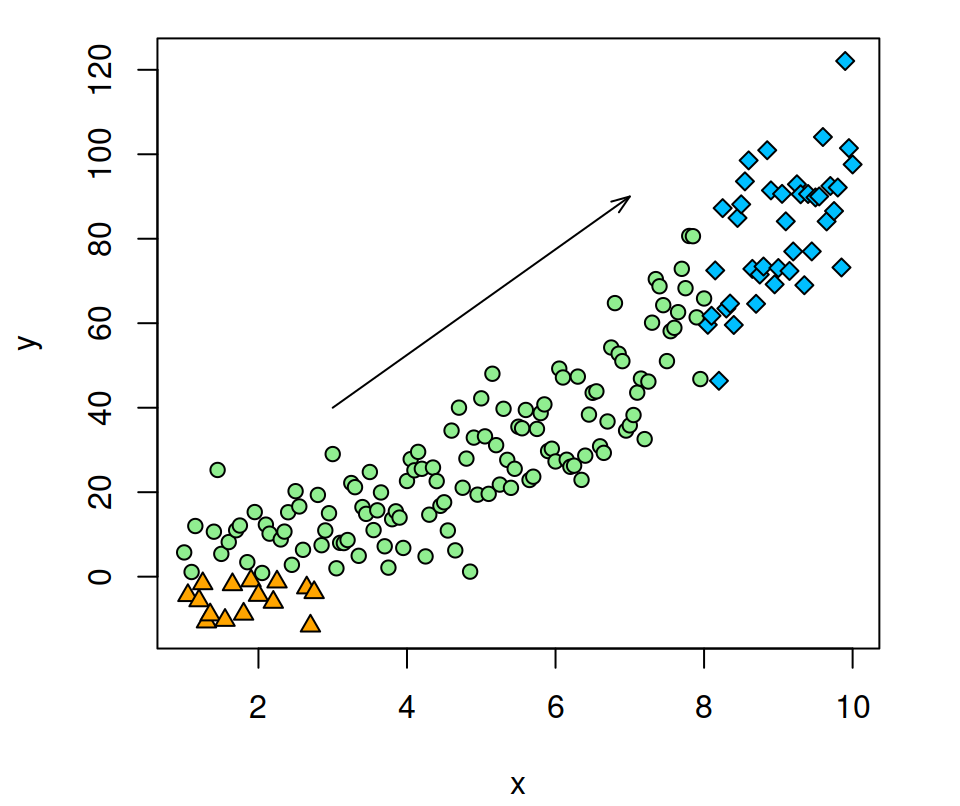# abline, segments and arrows

Base R provides several functions to add segments and arrows to the plots. In this guide we are going to use the following sample plot:

``````# Sample data
set.seed(132)
x <- seq(1, 10, by = 0.05)
y <- x ^ 2 + rnorm(x, sd = 10)

# Plotting function used in the examples below
splot <- function(x, y) {
plot(x, y, pch = ifelse(x > 8, 23, ifelse(y < 0, 24, 21)),
bg = ifelse(x > 8, "deepskyblue", ifelse(y < 0 , "orange", "lightgreen")))
}``````

## The `abline` function

The `abline` function allows drawing lines, such as horizontal lines (`h` argument), vertical lines (`v` argument), lines based on a intersection and a slope (`a` and `b` arguments) or for plotting a regression line.

Horizontal line

The `h` argument allows you to set the Y-axis value where to draw a horizontal line.

``````splot(x, y) # Sample function

# Horizontal line at Y = 0
abline(h = 0)``````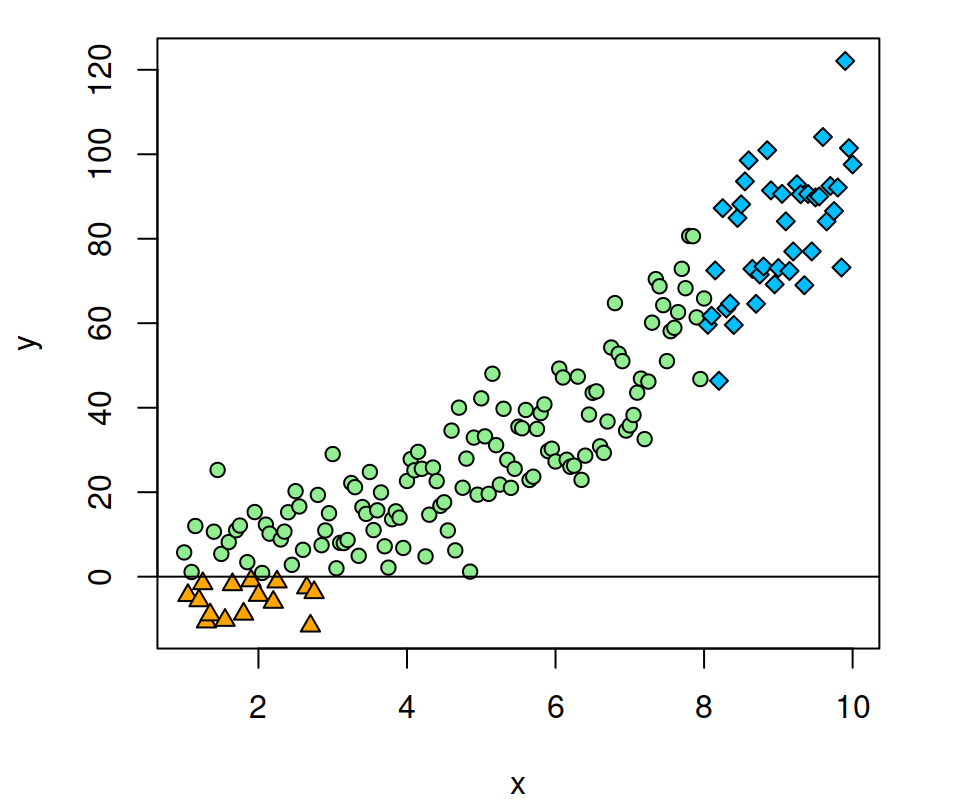Vertical line

The `v` argument allows you to set the X-axis values where to draw vertical lines.

``````splot(x, y)

# Vertical line at X = 8
abline(v = 8)``````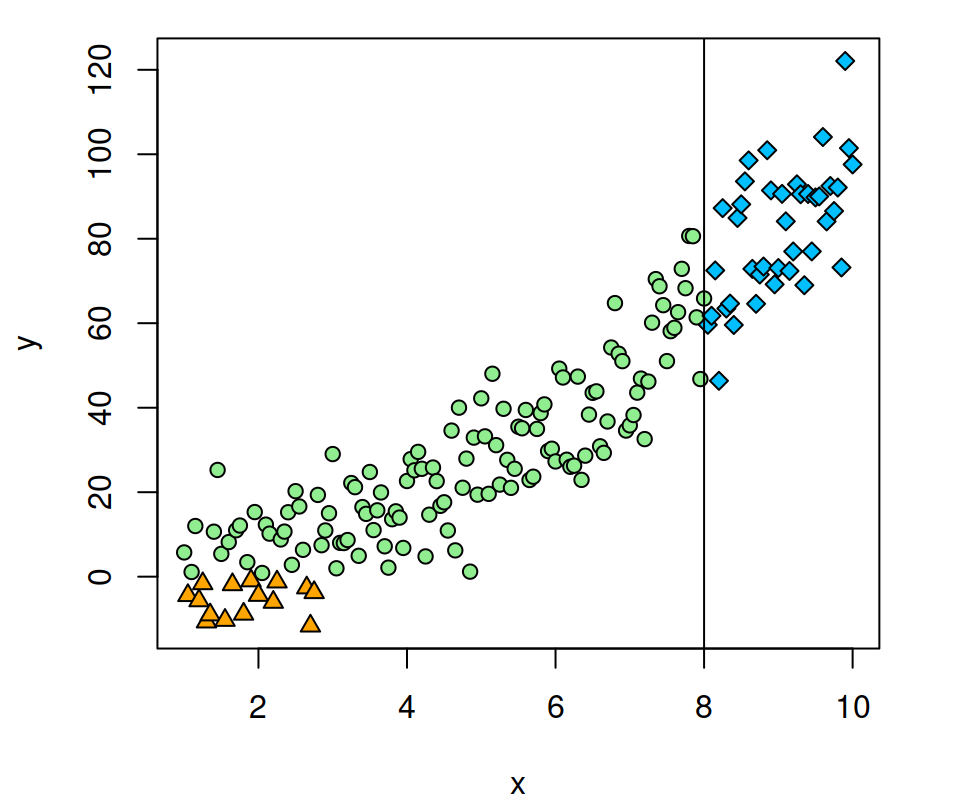Horizontal and vertical line at the same time

You can also add vertical and horizontal lines at the same time specifying both arguments.

``````splot(x, y)

# Horizontal line at Y = 1 and vertical at X = 8
abline(h = 1, v = 8)``````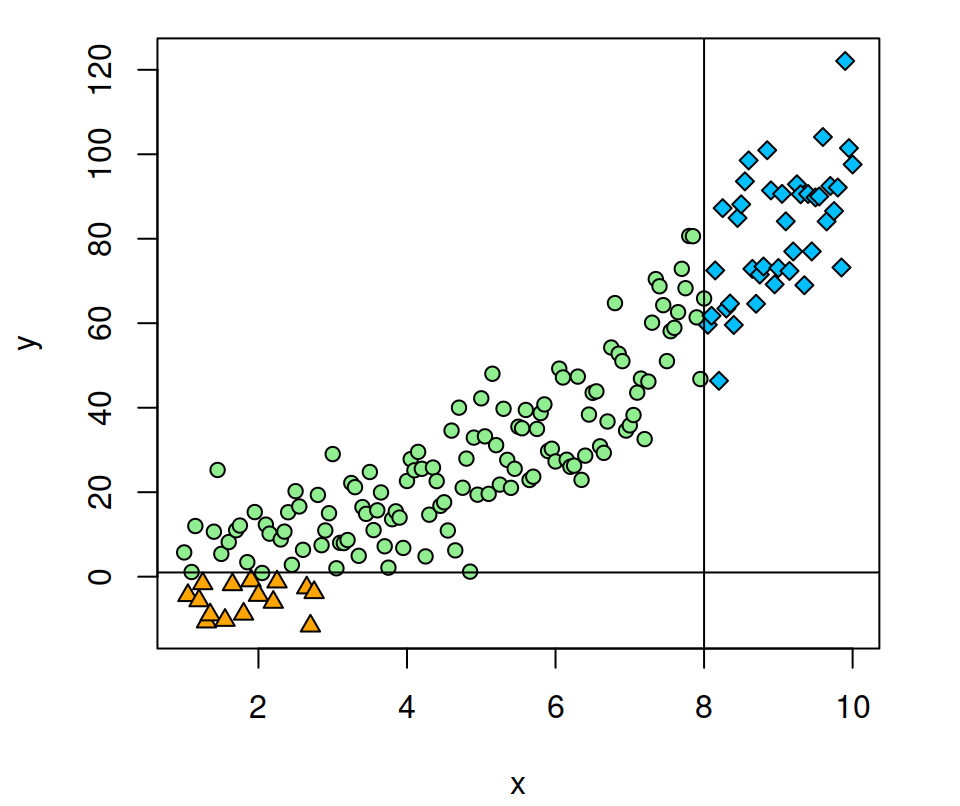Intercept and slope

In addition to horizontal or vertical lines, you can also specify an intercept with the argument `a` and the slope of the line with the argument `b`.

``````splot(x, y)

abline(a = -15, # Intercept
b = 10)  # Slope``````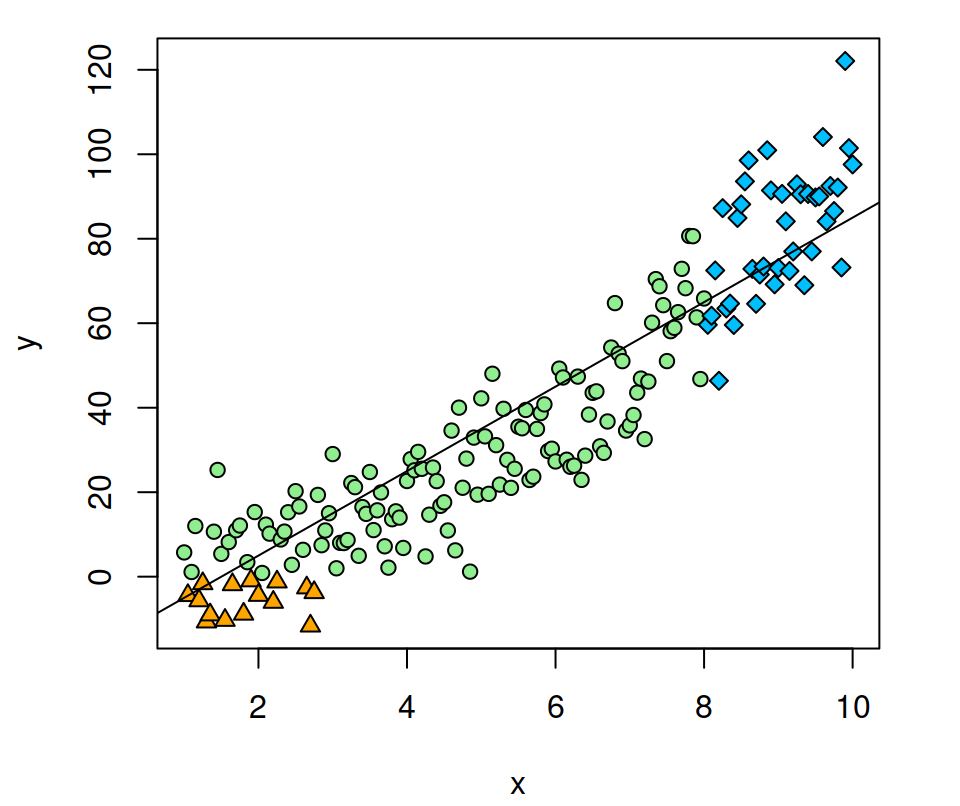Regression line

The `abline` function also allows drawing linear regression lines from a model.

``````splot(x, y)

abline(lm(y ~ x)) # Linear regression``````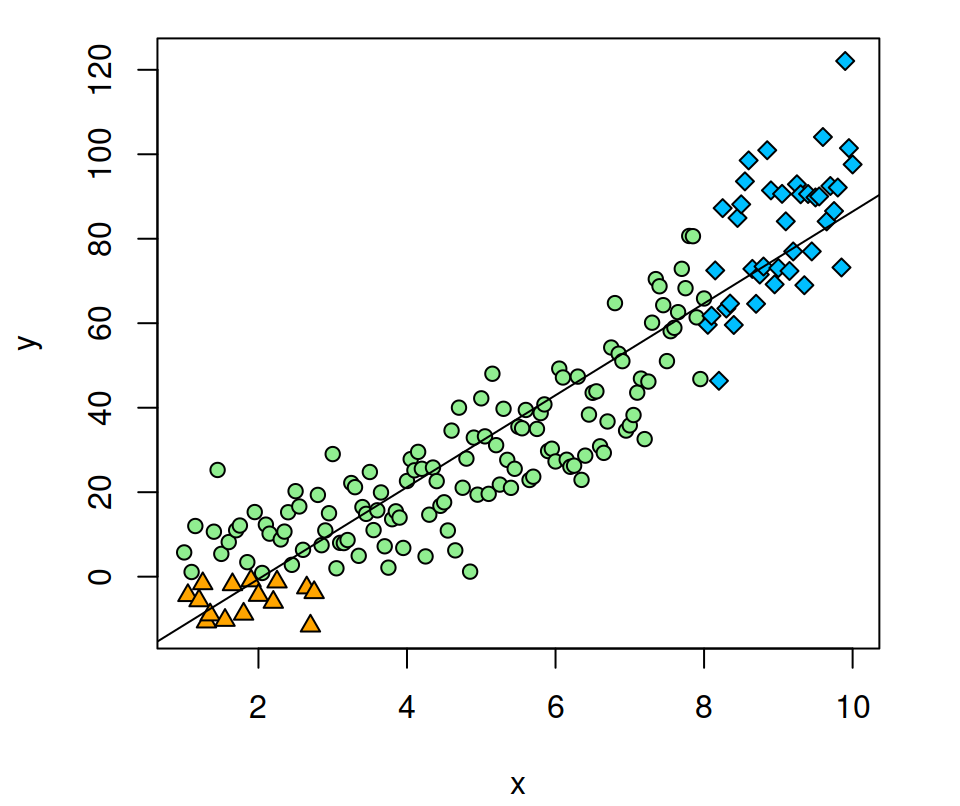Line customization

The same customization of lines in base R can be applied to this and the other functions of this guide.

``````splot(x, y)

abline(h = 0, v = 8,
col = c("red", "green"),
lwd = 2,
lty = 2:3)``````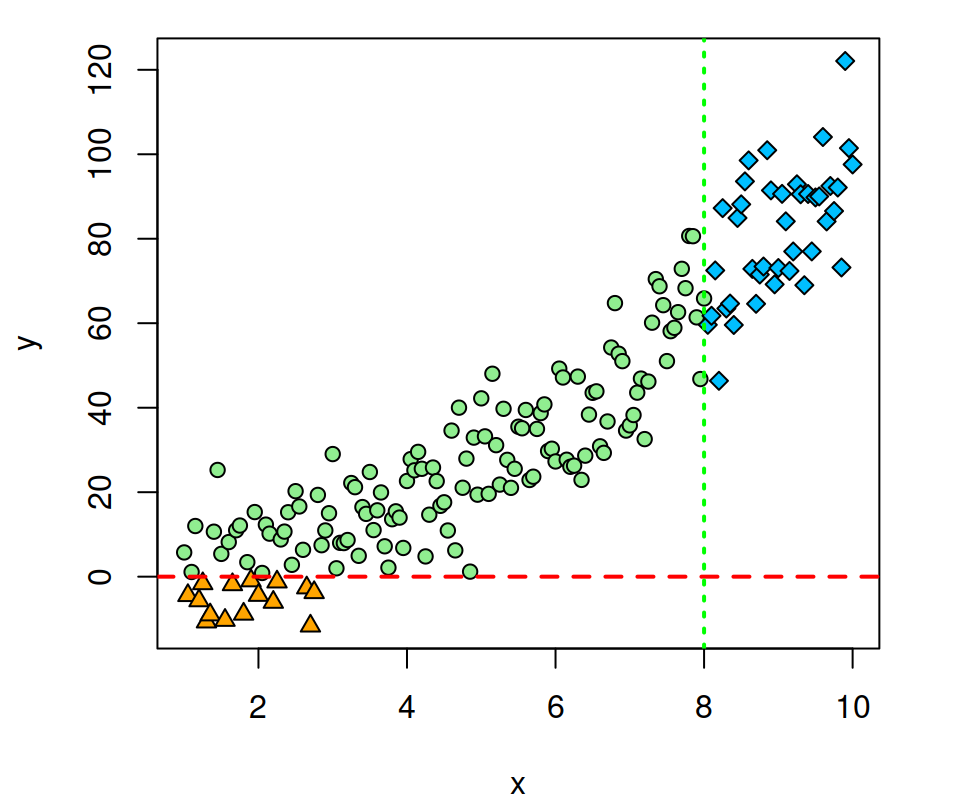## The `segments` function

This function is very similar to `abline`, but you can specify the starting and end points of the lines with `x0`, `x1`, `y0` and `y1` arguments.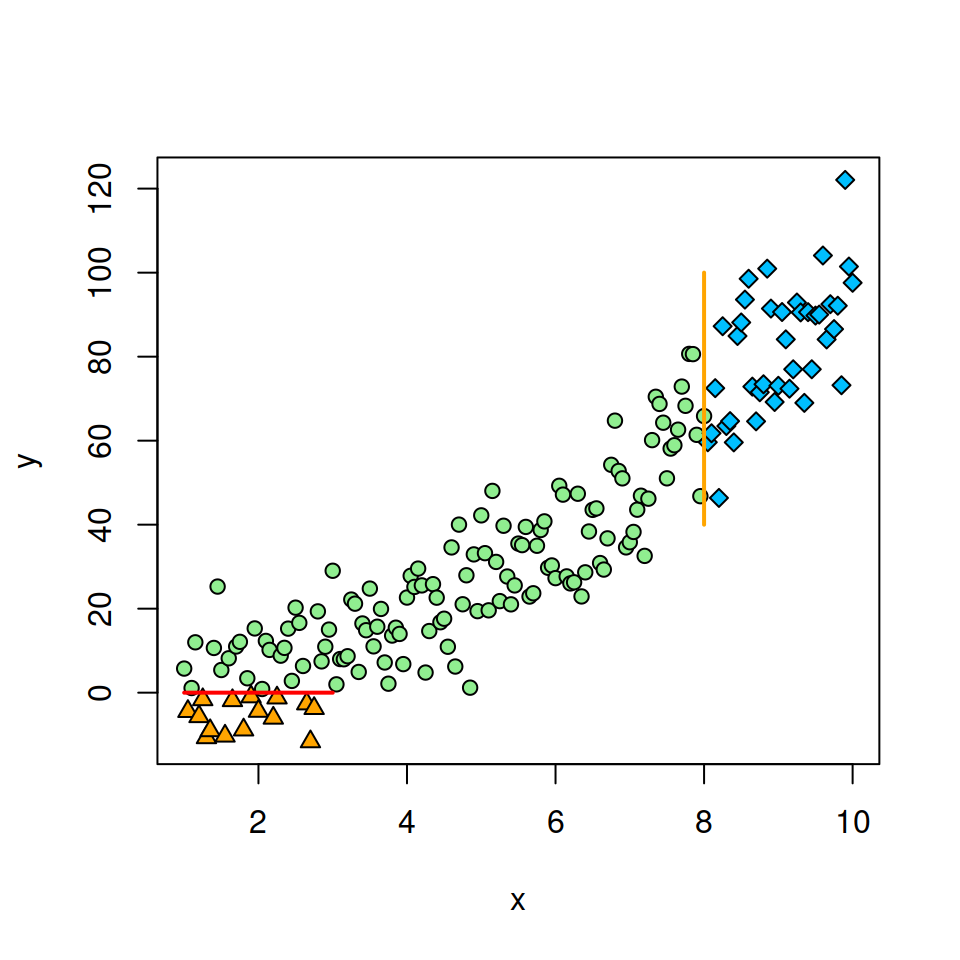``````splot(x, y)

segments(x0 = 1,
x1 = 3,
y0 = 0,
y1 = 0,
lwd = 2,
col = "red")

segments(x0 = 8,
x1 = 8,
y0 = 40,
y1 = 100,
lwd = 2,
col = "orange") ``````

## The `arrows` function

You can set the arrows the same way as segments, specifying the start and end of the arrow on each axis.

``````splot(x, y)

arrows(x0 = 3,
x1 = 7,
y0 = 40,
y1 = 90) ``````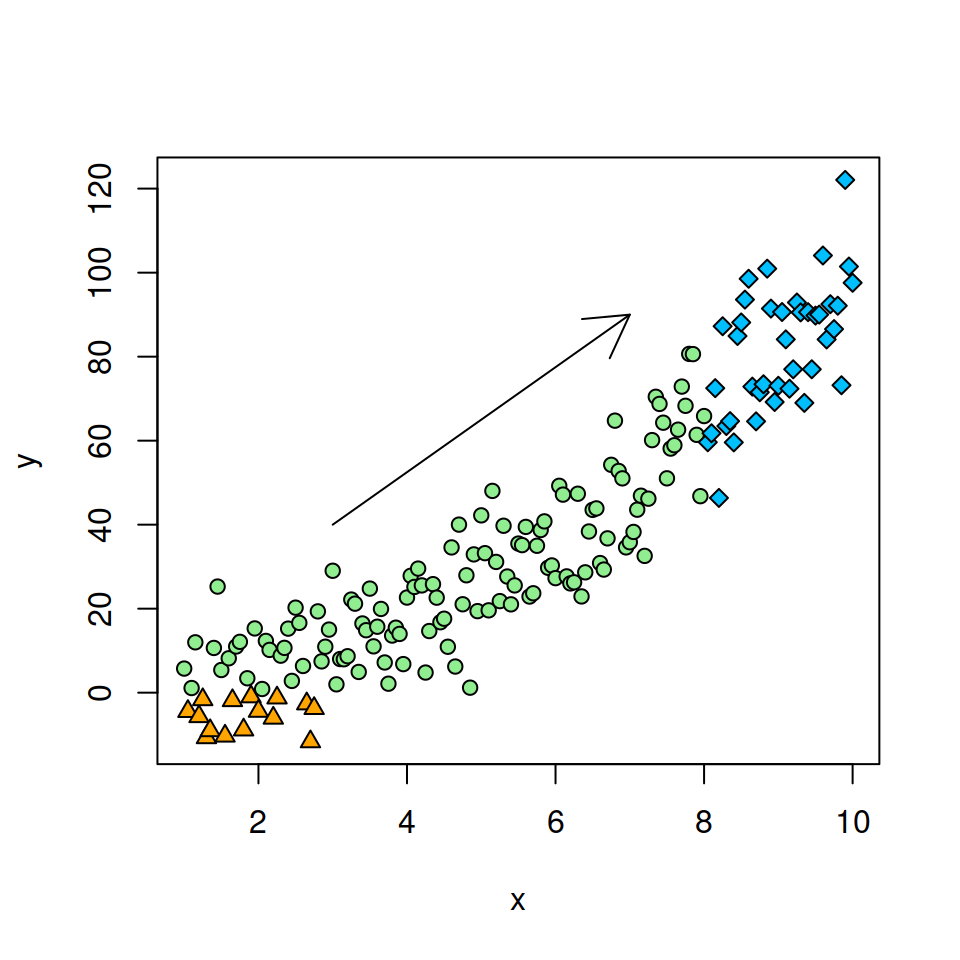In addition to modifying the color, width or line type, the `arrows` function also allows customizing the length and the angle of the arrowhead.

``````splot(x, y)

arrows(x0 = 3, x1 = 7,
y0 = 40, y1 = 90,
length = 0.1,
angle = 20)  ``````